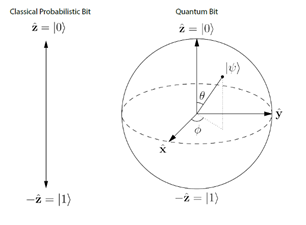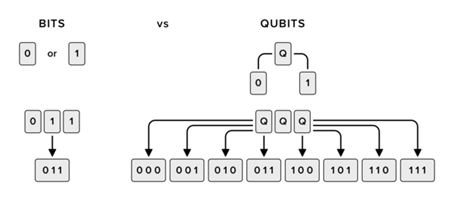# What can quantum physics contribute to computing?Authors:

Can you imagine a computer doing extraordinarily complex calculations, the kind that even just thinking about it already causes vertigo, while you calmly sip a cup of coffee? Well, maybe you don't have to imagine it that much.

A group of Chinese research centers, led by Jian-Wei Pan and Chao-Yang Lu, has created a quantum system called Jiuzhang - in honor of a practical manual on mathematics written between the 1st and 2nd centuries BCE in China - which has been capable of solving extremely complicated calculations in 200 seconds; milestone published by the Science magazine on December 18. To solve the same problem, a conventional supercomputer would have taken 2.5 billion years! To have a cup of coffee or to wait for evolution to go from bacteria to us. This timescale represents half the age of the Earth. Thus, the efficiency increases by a factor of 1014.

The calculations that the Chinese project undertakes to demonstrate the power of its quantum system correspond to a problem in particle physics known as "boson sampling." This pioneering quantum system that they have developed is characterized in that its qubits are photons and it works at room temperature. In contrast, in 2019 Google presented the 53-qubit Sycamore quantum computer, which, unlike the Jiuzhang quantum system, is programmable but has to work at temperatures close to absolute zero, -273.15 ° C. Despite the fact that the The Jiuzhang quantum system is not programmable and only solves a few specific calculations, if the problem is relevant enough, it may be interesting to build a machine specifically to tackle it. This Chinese project has demonstrated the usefulness of photonics to build quantum computers and has intensified this line of research.

To understand the enormous potential that quantum technology offers, it is important to present the qubit.

First, let us remember how the bit is defined. The bit - binary digit - is the minimum unit of information used in Computer Science and Information Theory. A bit can only have two mutually exclusive states that are commonly represented by 0 and 1.

The development of quantum mechanics allows us to establish a new unit of information: the qubit - quantum bit -. The possible states of the qubit are |0⟩ and |1⟩ following the Dirac notation, which is the standard notation for states in quantum mechanics, and are analogous to the 0 and 1 states of the classical bit. The difference between the qubit and the bit is that the qubit is a linear combination of both states |0⟩ and |1⟩, a fact that is called superposition.

|ψ⟩ = α |0⟩ + β |1⟩

The coefficients α and β are complex numbers (α, β ∈ ℂ) and determine with what probability the states |0⟩ and |1⟩ are obtained when a measurement is made. Thus, when we measure a qubit we obtain the value 0 with a probability |α|or the value 1 with a probability |β|2. Since the sum of the probabilities corresponding to all possible states is always 1, we obtain the following relationship: |α|+ |β|2 = 1.Figure 1. Graphical representation of the classical bit vs. the Bloch Sphere (representation of 1 qubit).

The superposition of states that the qubit introduces is extended when instead of having 1 qubit we have more. So, we observe that:

• 1 bit can be 0 or 1, while 1 qubit represents an overlap of 0 and 1.
• 2 bits can be 00, 01, 10 or 11, while 2 qubits represent an overlap of 00, 01, 10 and 11.
• 3 bits can be 000, 001, 010, 011, 100, 101, 110, or 111, while 3 qubits represent an overlap of 000, 001, 010, 011, 100, 101, 110, and 111.

And so on, so that a quantum system with N qubits represents 2states simultaneously.Figure 2. Representation of the classical Bit vs. Representation of the Qubit.

Therefore, on the one hand a classical system works following a Boolean logic and operates sequentially on N bits and, on the other hand, a quantum system follows a quantum logic that allows operating in parallel on 2N states.

In the same way that classical systems use the well-known logic gates - AND, OR, XOR, NOT -, quantum systems are governed by quantum gates. The nature of quantum gates is established by quantum mechanics and one of the most interesting properties is that, unlike the logic gates of classical systems, they are reversible.

This brief explanation captures some of the fundamental aspects of quantum computing that justify the great potential of the quantum revolution that we are experiencing. Currently, there are several initiatives dedicated to researching and developing emerging quantum technology. In the European Union, the Quantum Flagship project is underway, with funding of 1,000 million euros; in the United States there is a national quantum initiative, which has 1,200 million dollars, and China is building a huge laboratory of quantum technology and the national program counts on 15,000 million dollars.

In the future, with the help of quantum technology, great advances are expected in fields such as artificial intelligence, cybersecurity or the development of quantum neural networks, among other applications that we cannot even imagine right now due to the embryonic state in which this is.

Finally, it is convenient to remember the ephemeris of January 9, 1954, when the IBM company presented in New York the first integral circuit calculator or electronic brain, since it is possible that, right now, we are living a similar historical moment with all the advances in quantum technology that predict a hopeful future full of new possibilities.

BIBLIOGRAPHY / REFERENCES

Nielsen, M. A. & Chuang, I. L. (2012). Quantum Computation and Quantum Information. Cambridge: Cambridge University Press.

Zhong HS, Wang H, Deng YH, Chen MC, Peng LC, Luo YH, Qin J, Wu D, Ding X, Hu Y, Hu P, Yang XY, Zhang WJ, Li H, Li Y, Jiang X, Gan L, Yang G, You L, Wang Z, Li L, Liu NL, Lu CY, Pan JW (2020). Quantum computational advantage using photons. Science 370(6523):1460-1463.

Arute F, Arya K, Babbush R, Bacon D, et al. (2019). Quantum supremacy using a programmable superconducting processor. Nature. 574(7779):505-510.